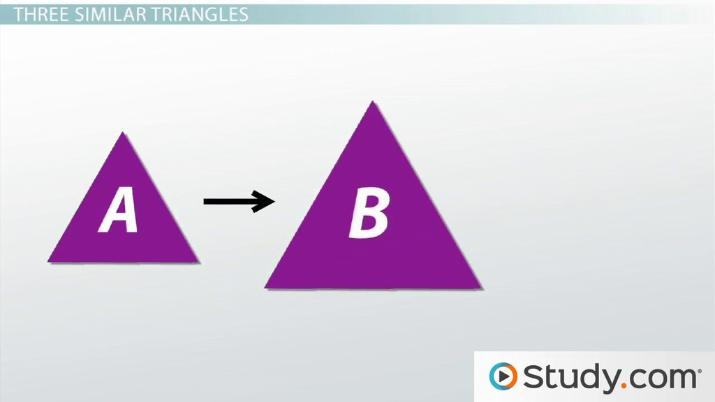Math Courses / Course / Chapter

# The Transitive Property of Similar Triangles

Lesson Transcript
Instructor: Yuanxin (Amy) Yang Alcocer

Amy has a master's degree in secondary education and has been teaching math for over 9 years. Amy has worked with students at all levels from those with special needs to those that are gifted.

The transitive property of similar triangles states that if triangle A is similar to triangle B, and triangle B is similar to triangle C, then triangle A is similar to triangle C, as well. Learn to use the transitive property of similar triangles to demonstrate that size is the only thing that is different about them.

## Similar Triangles

Triangles that are similar are triangles whose only difference is size. Similar triangles will look like they have either been shrunk or puffed up in size. Also, all the corresponding angles will be equal to each other. So, if the top angle of one triangle equals 45 degrees, then the top angles of all other similar triangles will also equal 45 degrees. If we compare the side lengths of one triangle to another, we will find that all the sides are proportional. So if the left side of one triangle compared to the left side of another triangle is twice as big, then all the other sides of the one triangle will also be twice as big as the corresponding sides of the other triangle. For example, if the left side of triangle A is 6 and the left side of triangle B is 3, then if the right side of triangle B is 4, then triangle A's right side must be 8 to maintain the proportion.An error occurred trying to load this video.

Try refreshing the page, or contact customer support.

Coming up next: Triangle Proportionality Theorem

### You're on a roll. Keep up the good work!

Replay
Your next lesson will play in 10 seconds
• 0:06 Similar Triangles
• 1:16 Transitive Property
• 2:09 3 Similar Triangles
• 2:45 Applying Transitive Property
• 3:57 Lesson Summary
Save Timeline
Autoplay
Autoplay
Speed Speed

## Transitive Property

The transitive property states that if a=b and b=c, then a=c. So, by the transitive property, I can say that if Joe is a boy and boys are tall, then Joe is tall. The transitive property helps you connect pieces of information together.

In algebra the transitive property is used to help you solve problems. If you had two different expressions that both equaled the same thing, then you can use the transitive property to help you connect your different expressions. For example, if you had x = 3 + 4y and you had 3 + 4y = z, then you can apply the transitive property and make the connection that x = z.

## Three Similar Triangles

In geometry, you can apply the transitive property to similar triangles to make connections. Let's see how this works. We start out with three triangles. We draw triangle A and then we draw a triangle B that is similar to triangle A. We then take triangle B and then we draw a triangle C that is similar to triangle B. So now we have three triangles: triangle A, triangle B, and triangle C. We know that triangle A is similar to triangle B and that triangle B is similar to triangle C.

To unlock this lesson you must be a Study.com Member.

### Register to view this lesson

Are you a student or a teacher?

Back

### Resources created by teachers for teachers

Over 30,000 video lessons & teaching resources‐all in one place.Video lessonsQuizzes & WorksheetsClassroom IntegrationLesson Plans

I would definitely recommend Study.com to my colleagues. It’s like a teacher waved a magic wand and did the work for me. I feel like it’s a lifeline.

Jennifer B.
TeacherCreate an account to start this course today
Used by over 30 million students worldwide